NCERT Solutions for Class 6 Maths Chapter 7 Fractions Ex 7.1 are part of NCERT Solutions for Class 6 Maths. Here we have given NCERT Solutions for Class 6 Maths Chapter 7 Fractions Ex 7.1.

 Board CBSE Textbook NCERT Class Class 6 Subject Maths Chapter Chapter 7 Chapter Name Fractions Exercise Ex 7.1 Number of Questions Solved 11 Category NCERT Solutions

## NCERT Solutions for Class 6 Maths Chapter 7 Fractions Ex 7.1

Question 1.
Write the fraction representing the shaded portion.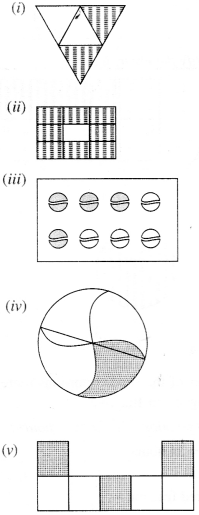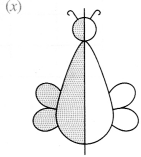Solution :Question 2.
Color the part according to the given fraction.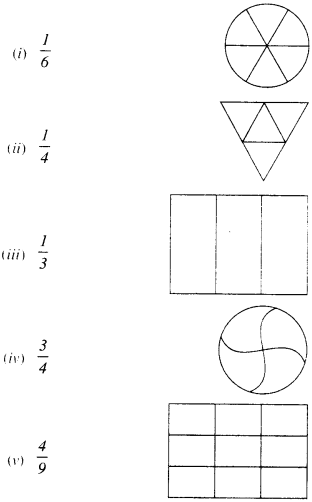Solution :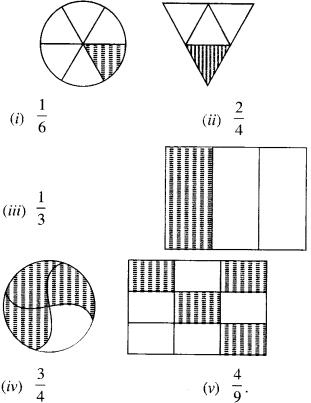Question 3.
Identify the error, if any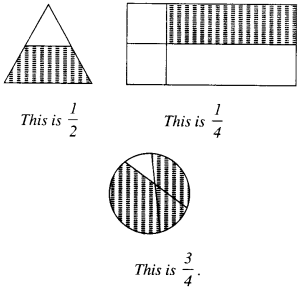Solution :
Neither of the shaded portions represents the corresponding given fractions. 4.

Question 4.
What fraction of a day is 8 hours?
Solution :
1 day = 24 hours
∴ Required fraction = $$\frac { 8 }{ 24 }$$

Question 5.
What fraction of an hour is 40 minutes?
Solution :
1 hour = 60 minutes
∴ Required fraction = $$\frac { 40 }{ 60 }$$

Question 6.
Arya, Abhimanyu, and Vivek shared lunch. Arya brings two sandwiches, one made of vegetable and one of jam. The other two boys forgot to bring their lunch. Arya agreed to share his sandwiches so that each person will have an equal share of each sandwich.
(a) How can Arya divide his sandwiches so that each person has an equal share?
(b) What part of a sandwich will each boy receive?
Solution :
(a) Arya will divide each sandwich into three equal parts, and give one part of each sandwich to each one of them.
(b) Each boy will receive $$\frac { 1 }{ 3 }$$ part of a sandwich.

Question 7.
Kanchan dyes dresses. She had to dye 30 dresses. She has so far finished 20 dresses. What fraction of dresses has she finished?
Solution :
She has finished $$\frac { 2 }{ 3 }$$ fraction of the dresses.

Question 8.
Write the natural numbers from 2 to 12. What fraction of them are prime numbers?
Solution :
The natural numbers from 2 to 12 are 2, 3, 4,5,6, 7, 8, 9, 10, 11 and 12
Total number of natural numbers = 11
Out of these, the prime numbers are 2, 3, 5, 7, 11

Total number of these prime numbers = 5 5
∴ Required fraction = $$\frac { 5 }{ 11 }$$.

Question 9.
Write the natural numbers from 102 to 113. What fraction of them are prime numbers?
Solution :
The natural numbers from 102 to 113 are
102, 103, 104, 105, 106, 107, 108, 109, 110, 111, 112 and 113
Total number of natural numbers =12
Out of these, the prime numbers are 103, 107, 109, 113.
Total number of these prime numbers = 4 . 4
∴ Required fraction = $$\frac { 4 }{ 12 }$$.

Question 10.
What fraction of these circles have X’s in them?Solution :
Total number of circles = 8
Number of circles which have X’s in them = 4
∴ Required fraction = $$\frac { 4 }{ 8 }$$.

Question 11.
Kristin received a CD player for her birthday. She bought 3 CDs and received 5 others as gifts. What fraction of her total CDs did she buy and what fraction did she receive as gifts?
Solution :
Number of CDs bought = 3
Fraction of her total CDs that she bought $$\frac { 3 }{ 8 }$$ and, fraction of her total CDs that she received as gifts $$\frac { 5 }{ 8 }$$.